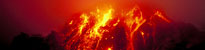Looking at data from several stationsSometimes it is useful to look at data from lots of different stations at the same time. The screenshot shows a 'record section'. It is based on data taken from several seismic monitoring stations following a large earthquake.

On a record section, the x-axis (time) is common for all traces, and the position of each trace up the page (the y-axis) depends on the distance of that recording station from the event.

Features to note

Study the sesimogram screenshot and note the features below:

• green marks show the arrival of direct P-waves
• orange marks show the arrival of direct S-waves
• red marks show the arrival of PP-waves (reflected at the surface)
• yellow marks show the arrival of ScS-waves (reflected at the boundary with the outer core)
• pink marks show the arrival of SS-waves (reflected at the surface)

Exercise

1. Which waves arrive first?
What do you notice about the difference in arrival times as the distance from the source increases?
2. Why do the P-wave and S-wave arrival times form curves instead of straight lines?
3. Choose two stations near the bottom of the seismogram and note down the arrival times of the P-wave and of the S-wave at each. Find the difference in time between arrival at one station and at the other for the P-wave and then for the S-wave.
4. Find the approximate difference in distance travelled from the source to each of these stations, assuming that 1 degree is equivalent to 111 km along the surface.
5. Use the distance difference and the time difference values you found to calculate an approximate speed for P-waves and for S-waves.
6. Repeat steps 3–5 for another pair of stations further up the seismogram, and compare the values you calculated for the speed of P-waves and S-waves.
7. Why might there be a difference? Why else might there be a difference?
8. The lines for the direct P-wave arrival (in green) and the direct S-wave arrival (orange) do not continue up to the furthest stations. What might be causing this?It is often useful to use a graph to let you read off values quickly without having to do a calculation. The graph uses the difference in arrival times of P-waves and S-waves to estimate the distance of a monitoring station from the source.

1. Why is the graph a curve, rather than a straight line?
2. Why does the x-axis stop at just beyond 100 degrees?
3. Use the time-travel graph and the same stations you used in the previous task to estimate their distances in degrees from the source. Compare the estimates with the values given on the y-axis.

Teachers' notesLooking at data from several stations

Students examine data from several stations at the same time, using a 'record section'.Earthquakes: how, why, where and when?
School seismology
FootballQuake
MarsQuake
News, teaching and museums